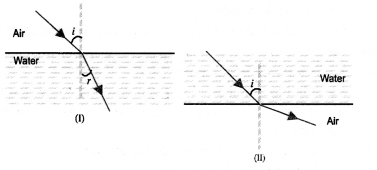## NCERT Exemplar Solutions for Class 10 Science Chapter 10 Light Reflection and Refraction

These Solutions are part of NCERT Exemplar Solutions for Class 10 Science. Here we have given NCERT Exemplar Solutions for Class 10 Science Chapter 10 Light Reflection and Refraction

Question 1.
Which of the following can make a parallel beam of light when light from a point source is incident on it ?
(a) Concave mirror as well as convex lens
(b) Convex mirror as well as concave lens
(c) Two plane mirrors placed at 90° to each other.
(d) Concave mirror as well as concave lens.
(a).
Explanation : When a point source is placed at the focus of a concave mirror or a convex lens, light after reflecting from the concave mirror and light after refracting from a convex lens goes as a parallel beam.

More Resources

Question 2.
A 10 mm long owl pin is placed vertically in front of a concave mirror. A 5 mm long image of the owl pin is formed at 30 cm in front of the mirror. The focal length of this mirror is
(a) – 30 cm
(b) – 20 cm
(c) – 40 cm
(d) – 60 cm
(b).Question 3.
Under which of the following conditions a concave mirror can form a real image larger than the actual object ?
(a) When object is kept at a distance equal to its radius of curvature.
(b) When object is placed between the focus and centre of curvature.
(c) When object is kept at a distance less than its focal length.
(d) When object is kept at a distance greater than its radius of curvature.
(b).
Explanation :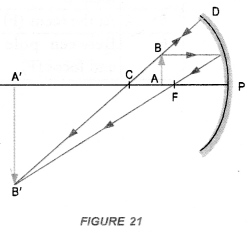Question 4.
Figure shows a ray of light as it travels from medium A to medium B. Refractive index of the medium B relative to medium A is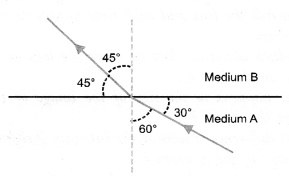(a).Question 5.
A light ray enters from medium A to medium B as shown in figure. The refractive index of medium B relative to medium A will be(a) greater than unity
(b) less than unity
(c) equal to unity
(d) zero
(a).
Explanation :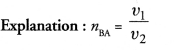where v1 = speed of light in medium A
V2 = speed of light in medium B.
Since ray of light bends towards the normal, when it goes from medium A to rrtedium B, therefore, medium A is rarer and medium B is denser medium. Speed of light in rarer medium (v1) is greater than the speed of light in denser medium (v2). Hence, nBA > 1.

Question 6.
Beams of light are incident through the holes A and B and emerge out of a box through the holes C and D respectively as shown in figure. Which of the following could be inside the box ?(a) A rectangular glass slab
(b) A convex lens
(c) A concave lens
(d) A prism.
(a).
Explanation : Rectangular glass slab causes the lateral dis¬placement of a ray of light following on it. However, incident ray and emergent ray are paralled to each other.

Question 7.
A beam of light is incident through the holes on side A and emerges out of the holes on the other side of the box as shown in figure. Which of the following could be inside the box ?(a) Concave lens
(b) Rectangular slab
(c) prism
(d) Convex lens
(d).
Explanation :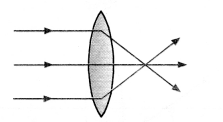Question 8.
Which of the following statements is true ?
(a) A convex lens has 4 dioptre power having focal length 0.25 m.
(b) A convex lens has – 4 dioptre power having focal length 0.25 m.
(c) A concave lens has 4 dioptre power having focal length 0.25 m.
(d) A concave lens has – 4 dioptre power having focal length 0.25 m.
(a).
Explanation : Convex lens has positive power and positive focal length.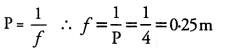Question 9.
Magnification produced by a rear view mirror fitted in vehicles.
(a) is less than one
(b) is more than one
(c) is equal to one
(d) can be more than or less than one depending upon the position of the object in front of it.
(a).Rear view mirror is a convex mirror, which always forms an image whose size is less than the size of the object.

Question 10.
Rays from sun converge at a point 15 cm in front of a concave mirror. Where should an object be placed so that size of its image is equal to the size of the object ?
(a) 15 cm in front of the mirror
(b) 30 cm in front of the mirror
(c) between 15 cm and 30 cm in front of the mirror.
(d) more than 30 cm in front of the mirror.
(b).
Explanation : Here, Focal length of concave mirror, f = -15 cm Radius of curvature of the mirror, R = 2f = -30 cm.
In case of concave mirror, size of image is equal to the size of the object if the object is placed at the centre of curvature (see figure 20). The distance of centre of curvature from the mirror = radius of curvature of the mirror.

Question 11.
A full length image of a distance tall building can definitely be seen by using
(a) concave mirror
(b) convex mirror
(c) plane mirror
(d) both concave as well as plane mirror.
(b).
Explanation : Convex mirror forms full length of a distant tall object irrespective of the position of the object. However, plane mirror forms full size image of the object if the size of the plane mirror is half the size of the object. Concave mirror forms full size image of the object if the object is far away from it.

Question 12.
In torches, search lights and head lights of vehicles, the bulb is placed
(a) between the pole and the focus of the reflector
(b) very near to the focus of the reflector
(c) between the focus and centre of curvature of the reflector
(d) at the centre of curvature of the reflector.
(b).
Explanation :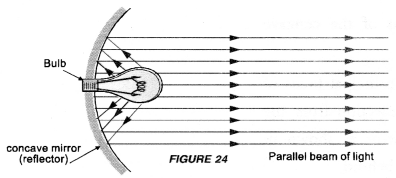Question 13.
The laws of reflection hold good for
(a) plane mirror only
(b) concave mirror only
(c) convex mirror only
(d) all mirrors irrespective of their shape
(d).

Question 14.
The path of a ray of light coming from air passing through a rectangular glass slab traced by four students are shown as A, B, C and D in the figure. Which one of them is correct ?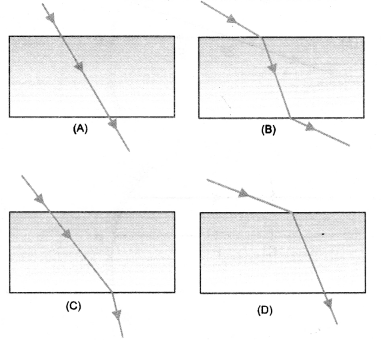(B)
Explanation : Glass slab causes the lateral displacement of a ray of light falling on it. However, incident ray and emergent ray are parallel to each other.

Question 15.
You are given water, mustard oil, glycerine and kerosene. In which of these media, a ray of light incident obliquely at same angle would bend the most ?
(a) Kerosene
(b) Water
(c) Mustard Oil
(d) Glycerine
(d).
Explanation : The ray would bend the most, when it goes from rarer medium (say air) to the most denser medium. Since refrac¬tive index of glycerine is the highest among all these medium, so glycerine is the most denser medium.

Question 16.
Which of the following ray diagrams is correct for the ray of light incident on a concave mirror as shown in figure ?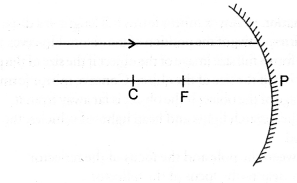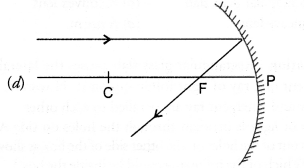(d).
Explanation : Any ray of light parallel to the principal axis passes through the focus (F) after reflecting from the concave mirror.

Question 17.
Which of the following ray diagrams is correct for the ray of light incident on a lens shown in figure ?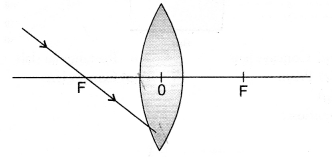(a).
Explanation : A ray of light passing through the focus of a lens travels parallel to the principal axis after refracting through the lens.

Question 18.
A girl is standing in front of a magic mirror. She finds the image of her head bigger, the middle portion of her body of the same size and that of the legs smaller. The order of combinations for the magic mirror from the top is :
(a) Convex, plane and concave
(b) Plane, convex and concave
(c) Concave, plane and convex
(d) Convex, concave and plane.
(c).
Explanation : Concave mirror forms a magnified (enlarged) image of the object if the object is placed close to the concave mirror (i.e. a distance less than its focal length). Plane mirror always forms the mage of the same size as that of the object.

Question 19.
Convex mirror always forms a diminished image of the object irrespective of the position of the object from the mirror. In which of the following, the image of an object placed at infinity will be highly diminished and point sized ?
(a) Concave mirror only
(b) Convex mirror only
(c) Convex lens only
(d) Concave mirror, convex mirror, concave lens and convex lens.
(d).
Explanation :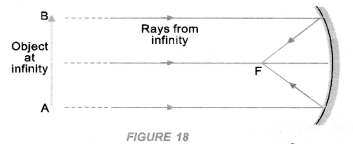Question 20.
Identify the device used as a spherical mirror or lens in the following cases, when the image formed is virtual and erect in each case.
(a) Object is placed between device and its focus, image formed is enlarged and behind it.
(b) Object is placed between the focus and device, image formed is enlarged and on the same side as that of an object.
(c) Object is placed between infinity and device, image formed is diminished and between focus and optical centre on the same side as that of the object.
(d) Obj ect is placed between infinity and device, image formed is diminished and between pole and focus, behind it.
(a) Concave mirror.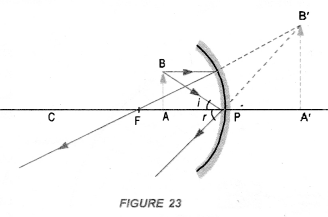(b) Convex lens.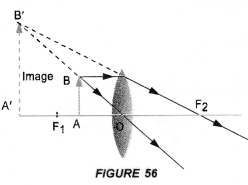(c) Concave lens.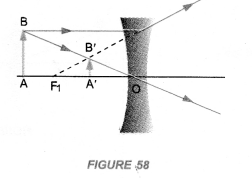(d) Convex mirror.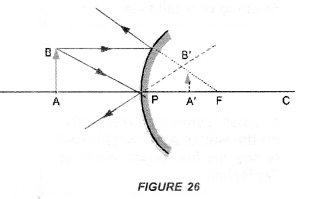Question 21.
Why does a light ray incident on a rectangular glass slab immersed in any medium emerges parallel to itself? Explain using a diagram.
Perform an experiment to demonstrate that light bends from its path, when it falls obliquely on the surface of a glass slab. Also show that angle of incidence is about equal to the emergent angle.

1. Take a glass slab and place it on a white sheet of paper fixed on a drawing board.
2. Mark the boundary ABCD of the glass slab.
3. Fix two pins P1 and P2, vertically on the drawing board such that line joining the pins is inclined to the edge AB of the glass slab (Figure 37).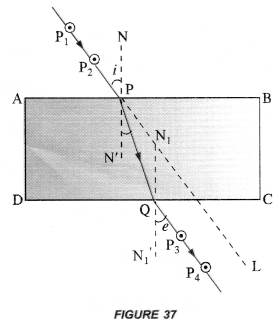4. Now, look through the glass slab from opposite side so that the images of pins P1 and P2 are seen exactly in line. Fix two pins P3 and P4, vertically on the drawing board such that pins P3, P4 and image of P1 and P2 are exactly in one line.
5. Remove the glass slab and all pins. Join the points P1 and P2 with a line and extend this line to touch the edge AB at P. Similarly join the points P3 and P4 with a line and extend the line to touch the edge DC at Q.
6. Join points P and Q with a straight line.
7. Draw normal NN’ at P on the edge AB and a normal N1N1‘ at Q on the edge DC.
8. Measure ∠P2 PN and ∠P3 QN1‘ using a protractor or dee.

This experiment shows that when light falls obliquely on a glass slab, it bends along PQ from its original path along PL.
Also ∠P2PN ≈ ∠P3QN1‘. That is, angle of incidence is about equal to the emergent angle.
Note : Distance between pins P1 and P2, and between P3 and P4 must be large.

Question 22.
A pencil when dipped in water in a glass tumbler appears to be bent at the interface of air and water. Will the pencil appear to be bent to the same extent, if instead of water, we use liquids like, kerosene or turpentine. Support your answer with reason.
A pencil dipped in water appears to be bent at the interface of air and water due to the refraction of light. The refraction of light occurs because the speed of light changes when light travels from one medium to another. The pencil will not appear to be bent to the same extent, when it is dipped in kerosene or turpentine. This is because refractive index of kerosene or turpentine is greater than the index of water and hence speed of light (v = c/n) is less in kerosene or turpentine as compared to in water.

Question 23.
How is the refractive index of a medium related to the speed of light ?
Obtain an expression for refractive index of a medium with respect to another in terms of speed of light in these two media.
Refractive index of a medium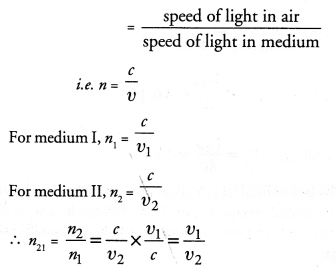Question 24.
Refractive index of diamond with respect to glass is 1.6 and absolute refractive index of glass is 1.5.
Find out the absolute refractive index of diamond.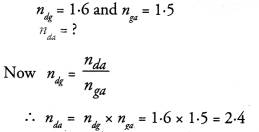Question 25.
A convex lens of focal length 20 cm can produce a magnified virtual as well as real image.
Is this a correct statement ? If yes, where shall the object be placed in each case for obtaining these images ?
The statement is correct.
A convex lens of focal length 20 cm will produce a magnified Virtual image if object is placed at a distance less than 20 cm from the lens.A convex lens of focal length 20 cm will produce a magnified real image if object is placed at a distance greater than 20 cm and less than 40 cm from the lens.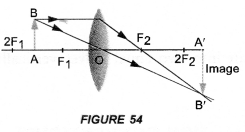Sudha finds out that the sharp image of the window pane of her science laboratory is formed at a distance of 15 cm from the lens. She now tries to focus the building visible to her outside the window instead of the window pane without disturbing the lens.

Question 26.
In which direction will she move the screen to obtain a sharp image of the building ? What is the approximate focal length of this lens ?
A real image can be obtained on the screen. Therefore, the lens used is convex lens as it forms real as well as virtual image. The distance of the real image formed by a convex lens from the lens decreases as the object distance from the lens increases. Hence, the screen has to be moved towards the lens to obtain the sharp image of the building.
Approximate focal length of the lens = 15 cm. The rays of light from the window pane are considered to come from infinity. These rays of light are focused by the convex lens at its focus (i.e. on the screen).

Question 27.
How are power and focal length of a lens related ? You are provided with two lenses of focal length 20 cm and 40 cm respectively. Which lens will you use to obtain more convergent light ? (CBSE 2012)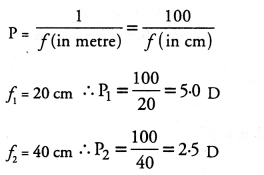The lens of focal length 20 cm or power 5.0 D will be used to have more convergent light. This is because lens of small focal length or large power strongly converges the parallel beam of light.

Question 28.
Under what condition in an arrangement of two plane mirrors, incident ray and reflected ray will always be parallel to each other, whatever may be the angle of incidence. Show the same with the help of diagram.
Incident ray and reflected ray will always be parallel to each other if two plane mirrors are placed perpendicular to each other as shown in figure, i = r.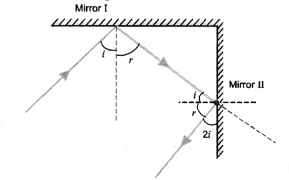Question 29.
Draw a ray diagram showing the path of rays of light when it enters with oblique incidence

1. from air into water,
2. from water into air.